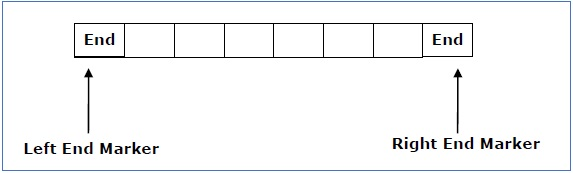# Linear Bounded Automata

A linear bounded automaton is a multi-track non-deterministic Turing machine with a tape of some bounded finite length.

Length = function (Length of the initial input string, constant c)

Here,

Memory information ≤ c × Input information

The computation is restricted to the constant bounded area. The input alphabet contains two special symbols which serve as left end markers and right end markers which mean the transitions neither move to the left of the left end marker nor to the right of the right end marker of the tape.

A linear bounded automaton can be defined as an 8-tuple (Q, X, ∑, q0, ML, MR, δ, F) where −

• Q is a finite set of states

• X is the tape alphabet

• is the input alphabet

• q0 is the initial state

• ML is the left end marker

• MR is the right end marker where MR ≠ ML

• δ is a transition function which maps each pair (state, tape symbol) to (state, tape symbol, Constant ‘c’) where c can be 0 or +1 or -1

• F is the set of final statesA deterministic linear bounded automaton is always context-sensitive and the linear bounded automaton with empty language is undecidable..